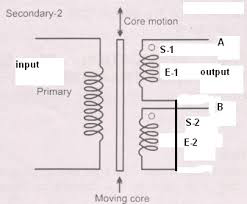# Working of LVDT (linear variable differential transformer)

As per the position of core, the secondary coils S1 and S2 both are produce emf which is phase. The lower end of both secondary coils S1 and S2 are joined together to get a differential output. According to the position of the core, three different conditions are formed.Condition 1:

When the core is at the center, both secondary coils (S1 and S2) produce equal emf. The two secondary coils (S1 and S2) are connected in phase opposition so that the differential output potential in above condition is zero

E1 = E2

E0 = E1-E2 = 0

Condition 2:

When the core is moved in upward direction i.e. towards S1, the emf induced at S1 is more as compared to S2. Therefore, the differential output potential is positive.

E1 > E2

E0 = E1-E2 = +ve (positive)

Condition 3:

When the core is move downward i.e. towards S2, the emf induced at S2 is more as compared to S1. Since, S2 produce output 180 degree out of phase. Therefore, the differential output potential is negative.

E1 < E2

E0 = E1 –E2 = negative

Tags: